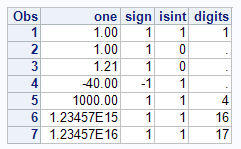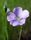## verify number and length

I have a numer of fields that I need to verify are a number with a length smaller than 17 and no decimal places.  Through my ETL I'm verifying it's a number, to verify length I'm using strip(length()), and to verify there are no decimal places I'm using scan().  Is there a more efficient way of doing this?

Thank You,

6 REPLIES 6

## Re: verify number and length

Well, to my mind if the string should only be max 17, then it would be best to set the length of that variable to 17 in the first place, this check would be irrelevant then.

You could trim your code a little bit by using the lengthn() function rather than strip(length()).

Other than that I don't have much else to offer, the metadata of the file refers to what strcuture has been set, not a length of data, which is why I recommend the strcuture matches what is expected.

## Re: verify number and length

It's not a string, I have to verify it's a number with a length of 16.0.  The number part will be a verified at load time, I'm using the length function to verify the length and the scan function to make sure there are no numbers after a decimal point.

## Re: verify number and length

What about substracting the maximum value possible for 16 numbers from the number and seeing if it is greater than 0:

```data tmp;
num=1234567890123456.0;
if num-9999999999999999 > 0 then result="Yes"; output;
num=12345678901234567.0;
if num-9999999999999999 > 0 then result="Yes"; output;
run;```

## Re: verify number and length

Here's is what I have that I like better than my original code:

data data;
informat one 16.;
format one 16.;
input one;
cards;
1
1.21
1000
1234567891123456
12345678911234567
;run;

data qc;
set data;
if not missing(scan(one,2,'.')) then check = 1;
else check = 0;
run;

the etl will only load a number, the long number goes to 1.E so scan will pick it up, the decimal will get picked up using the scan also.  I don't like that the 1.21 doesn't show in the output but it flips the error so I think it's acceptable.data_null__

## Re: verify number and length

It sounds like the rule is positive integer with LE 16 digits.

``````data data;
input one;
sign  = sign(one);
isint = int(one) eq one;
if isint and sign then digits = 1+int(log10(one));
cards;
1
1.000000001
1.21
-40
1000
1234567891123456
12345678911234567
;;;;
run;
proc print;
run;``````LinusH
Tourmaline | Level 20

## Re: verify number and length

What do you mean by efficient?

These are two sets of SAS function operations. Can'r see any performance problem there...?

You could probably use regular expressions, some thinks it's neater, and maybe it is. But perhaps not as readable as the SAS function counterparts.

Data never sleeps
Discussion stats
• 6 replies
• 1071 views
• 0 likes
• 4 in conversation